# One ball

One ball of dough can be stretched into a circle to make a pizza. After the pizza is cooked, it is cut into 8 equal slices. How many slices of pizza can you make with 3 balls of dough? Solve this problem any way you choose.

n =  24

### Step-by-step explanation: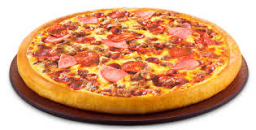Did you find an error or inaccuracy? Feel free to write us. Thank you!## Related math problems and questions:

• Pumpkin pie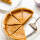Have some pumpkin pie. One half of the pie is cut into 4 equal slices, the other half is cut into 3 equal slices. After eating one of the larger slices (on the 3 piece side), I wonder if I will eat more if I have one more from the 3 side, or two from the
• Slices of pizzaMaria ate 1/4 of a pizza. If there were 20 slices of pizza, how many slices did Maria eat?
• White balls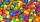I have 3 white balls and 5 red balls in my pocket. How many least balls do I have to remove from the bag to make sure that the pulled out ball is definitely red?
• A pizza 2A pizza is cut into slices that are each 1/6 of the whole. John is going to eat 1/2 of the whole pizza. How many slices will John eat?
• The dough 2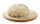A baker made 8 pounds of dough. He used 5/9 of the dough to make sandwich rolls. How much of the dough is left over?
• CindyCindy bought four slices of pizza for Php 95.60. Whole pizza has 6 slices. How much does each slice cost?
• Balls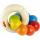The boys changed stamps, beads and balls. For 9 balls is 3 stamps, 2 balls is 44 stamps. How many beads is for 1 ball?
• White and black ballsThere are 7 white and 3 black balls in an opaque pocket. The balls are the same size. a) Randomly pull out one ball. What is the probability that it will be white? We pull out one ball, see what color it is and return it to the pocket. Then we pull out th
• Balls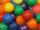I have 19 balls and my friend has 27. About how many has my friend more balls?
• Billiard ballsA layer of ivory billiard balls of radius 6.35 cm is in the form of a square. The balls are arranged so that each ball is tangent to every one adjacent to it. In the spaces between sets of 4 adjacent balls other balls rest, equal in size to the original.
• A boxA box contains 6 red balls, 5 blue, and 4 white balls. Find the probability of drawing a) red ball b) non-white ball.
• Balls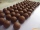From the bag with numbered balls (numbers 1,2,3,. ..20) we pick one ball. What is the probability of choosing a number containing 1?
• Write 3Write a real-world problem involving the multiplication of a fraction and a whole number with a product that is between 8 and 10, then solve the problem.
• Balls groups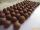Karel pulled the balls out of his pocket and divided them into groups. He could divide them in four, six, or seven, and no ball ever left. How little could be a ball?
• Pizza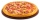Five friends were together for pizza. Adam divided his pizza into thirds, Boris in quarters, Denis in patina and Luke in sixth. Then Simon also came to them. Each of the five boys gave him one piece, leaving him one whole pizza. In how many equal parts di
• Balls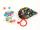Michal said to Martin: give me one ball, and I'll have twice as you. Martin said: give me 4, and we will have equally. How many balls each have?
• Plasticine ballPlasticine balls have radius r1=85 cm, r2=60 mm, r3=59 cm, r4=86 cm, r5=20 cm, r6=76 mm, r7=81 mm, r8=25 mm, r9=19 mm, r10=14 cm. For these balls